# Question 1. Consider these real-valued functions of two variables: T In (x2 + y2) (a) () What is ...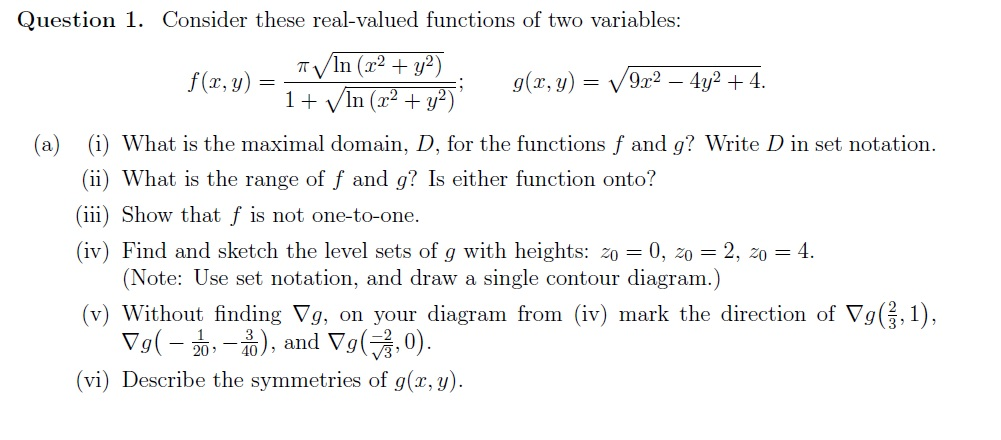Question 1. Consider these real-valued functions of two variables: T In (x2 + y2) (a) () What is the maximal domain, D, for the functions f and g? Write D in set notation. (ii) What is the range of f and g? Is either function onto? ii) Show that f is not one-to-one. (iv) Find and sketch the level sets of g with heights: z00, 2, 04 (Note: Use set notation, and draw a single contour diagram.) (v) Without finding ▽g, on your diagram from (iv) mark the direction of ▽g(3,1). and Vg 2,0 20 40 (vi) Describe the symmetries of g(x, y)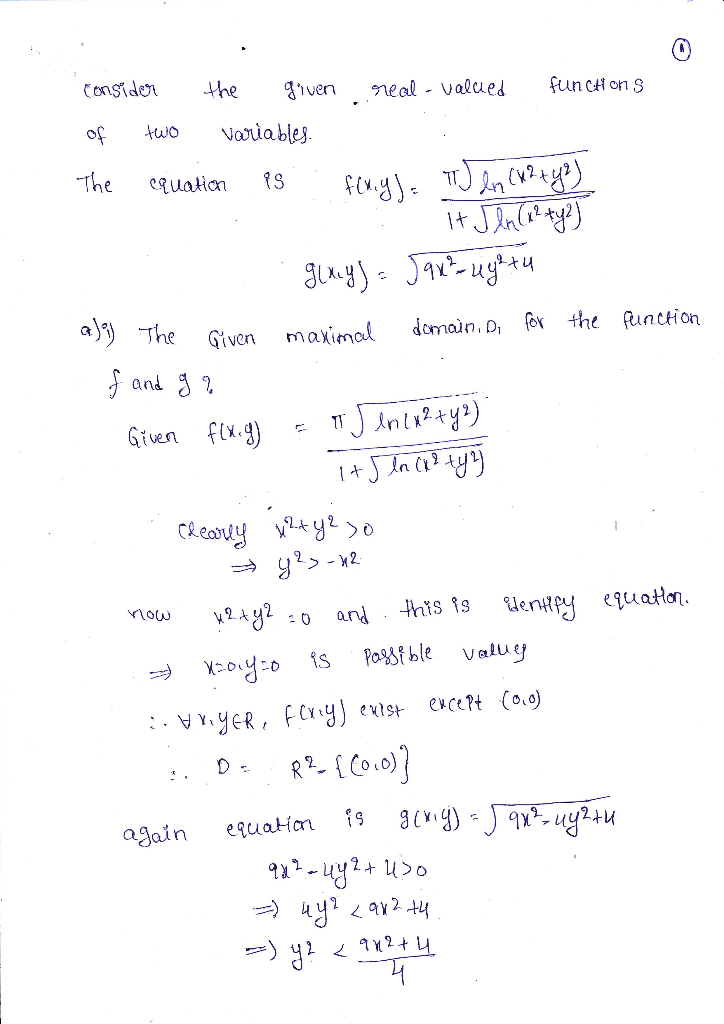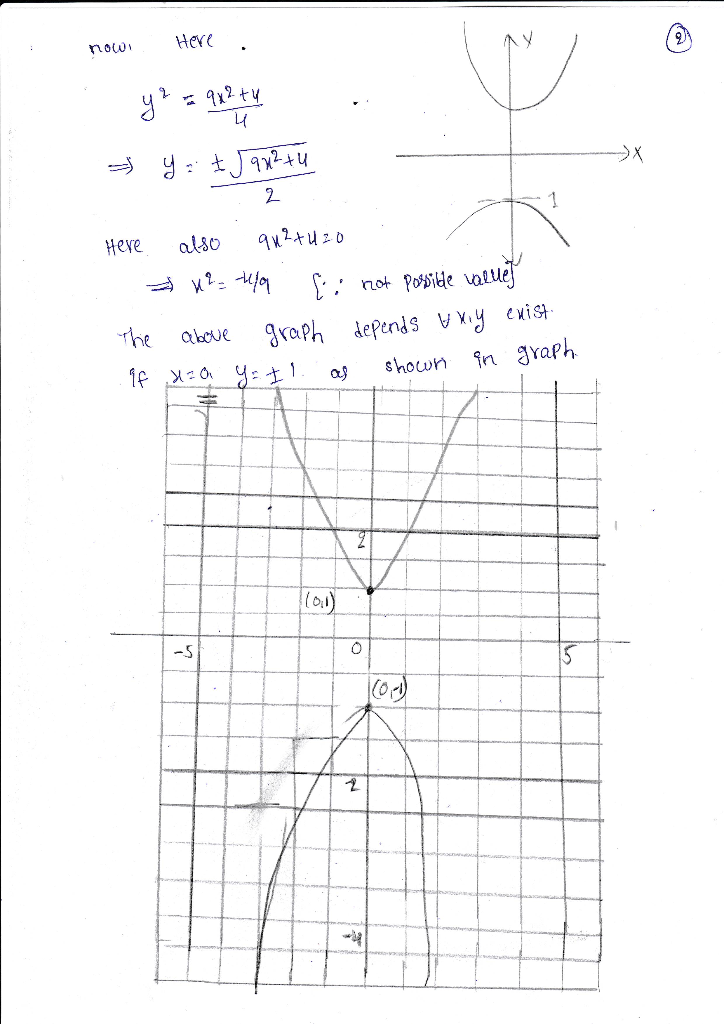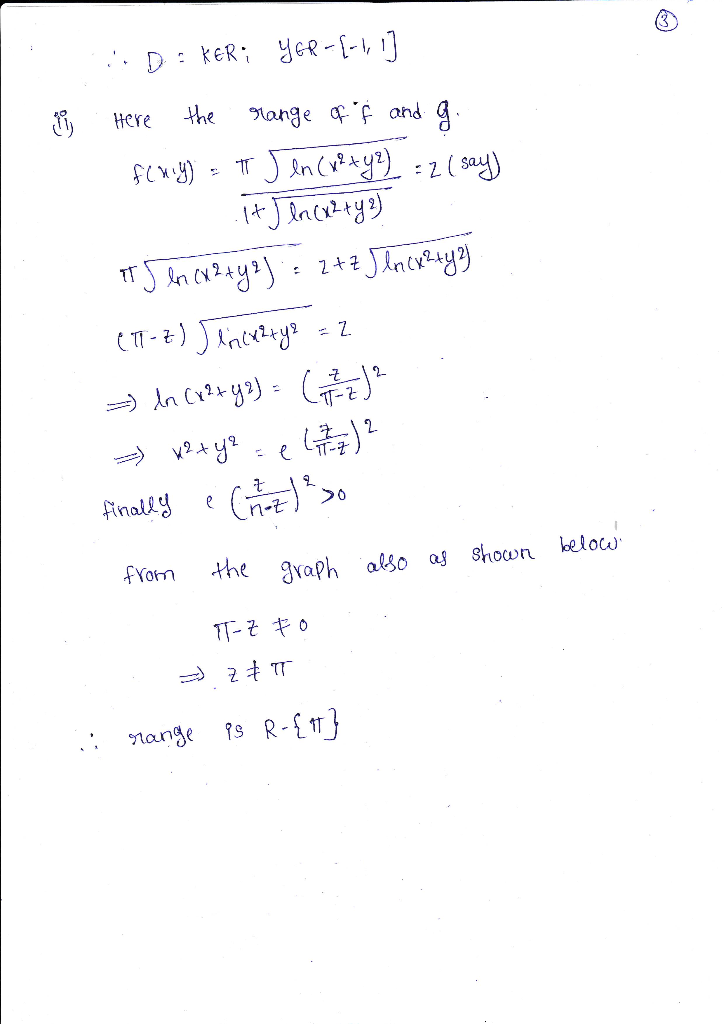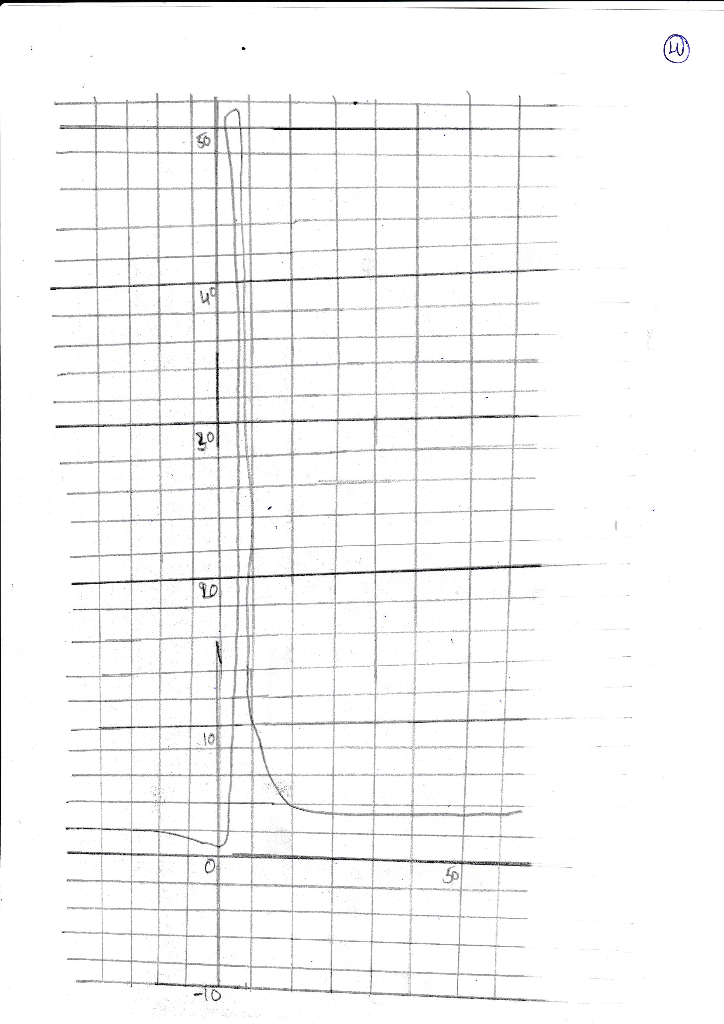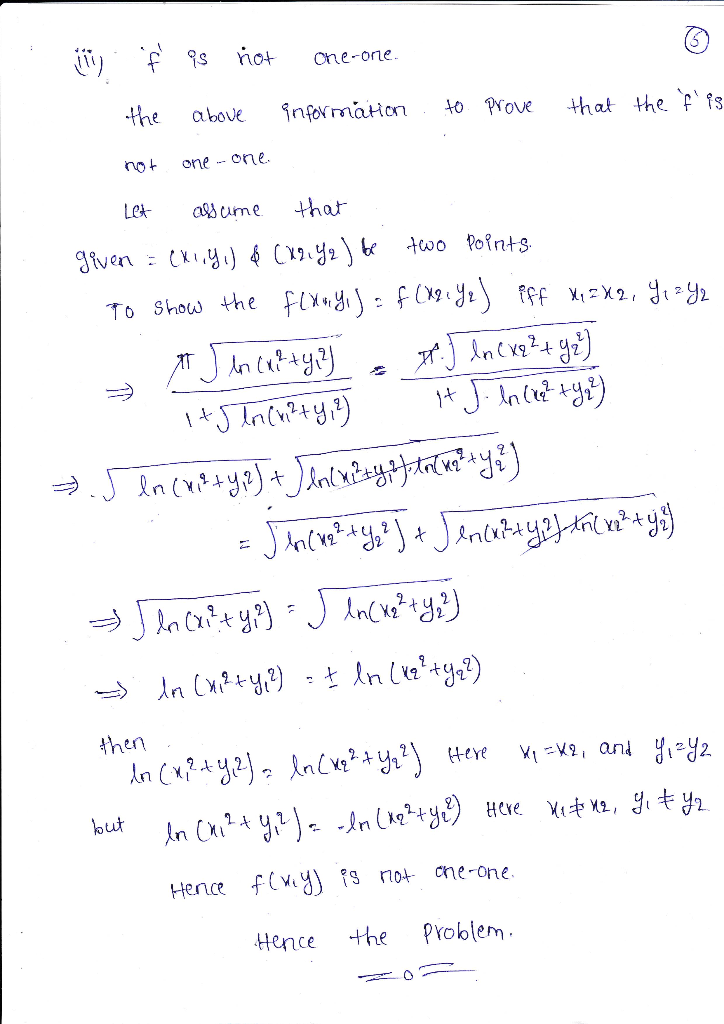#### Earn Coin

Coins can be redeemed for fabulous gifts.

Similar Homework Help Questions
• ### Question 1. Consider these real-valued functions of two variables: (a) i) What is the maximal dom...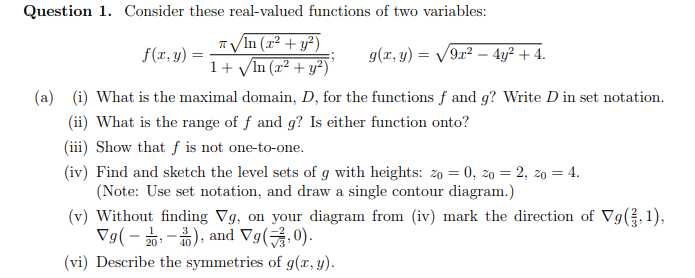Question 1. Consider these real-valued functions of two variables: (a) i) What is the maximal domain, D, for the functions f and g Write D in set notation (ii) What is the range of f and g? Is either function onto? ii) Show that f is not one-to-one iv) Find and sketch the level sets of g with heights: zo- 0, 0 2, 20 4 Note: Use set notation, and draw a single contour diagram.) v) Without finding Vg, on...

• ### Question 1. Consider these real-valued functions of two variables f(x, y) (a) (i) What is the max...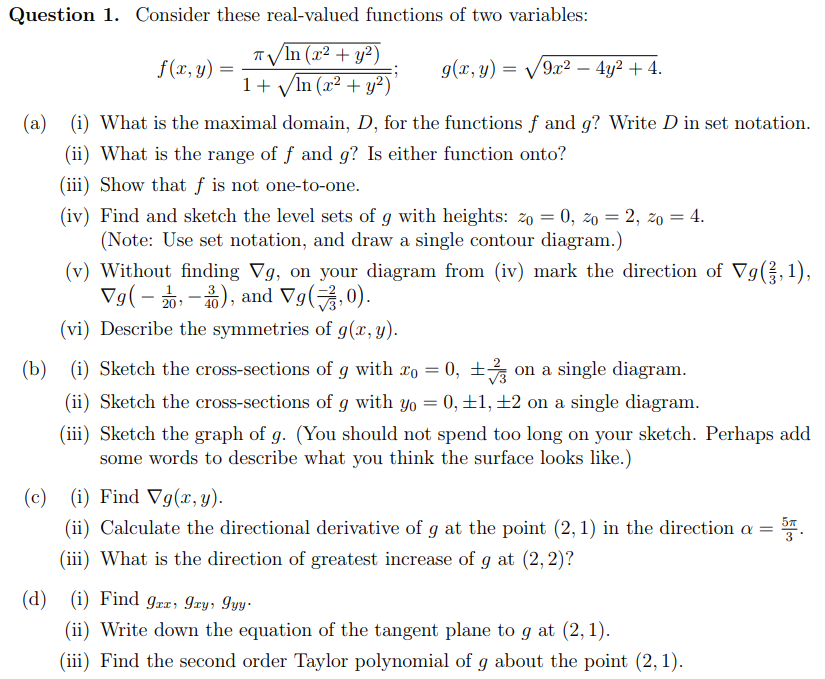Question 1. Consider these real-valued functions of two variables f(x, y) (a) (i) What is the maximal domain, D, for the functions f and g? Write D in set notation (ii) What is the range of f and g? Is either function onto? (iii) Show that f is not one-to-one. (iv) Find and sketch the level sets of g with heights: 20-0, 20-2, 20-4 (Note: Use set notation, and draw a single contour diagram.) (v) Without finding Vg, on your...

• ### Question 1. Consider these real-valued functions of two variables TVIn (r2y2) f (x, y)- 9(r,)2 2+...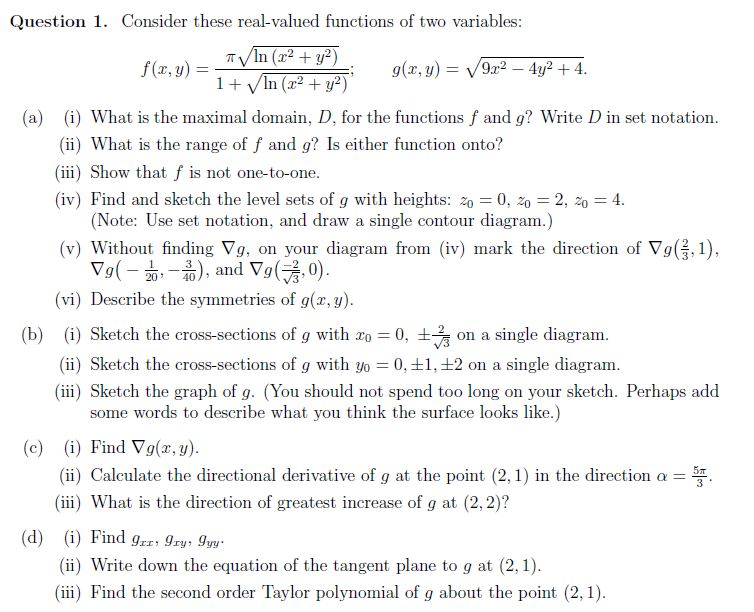Question 1. Consider these real-valued functions of two variables TVIn (r2y2) f (x, y)- 9(r,)2 2+4 (a) (i) What is the maximal domain, D, for the functions f and g? Write D in set notation (ii) What is the range of f and g? Is either function onto? iii) Show that f is not one-to-one. (iv) Find and sketch the level sets of g with heights: z0 0, 20 2, 204 (Note: Use set notation, and draw a single contour...

• ### Question 1. Consider these real-valued functions of two variables: +in (b) (i) Sketch the cross-s...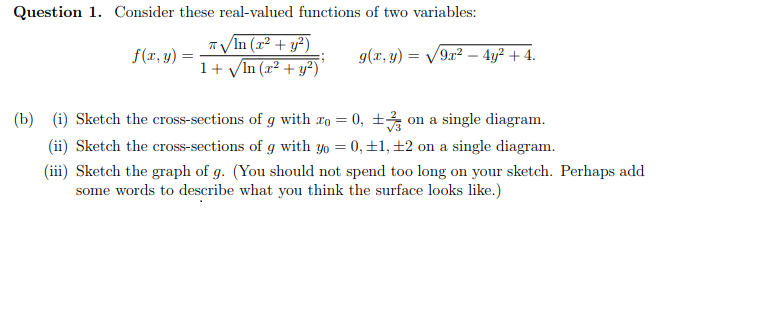Question 1. Consider these real-valued functions of two variables: +in (b) (i) Sketch the cross-sections of g with 20-0, ±v3 on a single diagram. (ii) Sketch the cross-sections of g with yo 0, +1, 2 on a single diagram (ii) Sketch the graph of g. (You should not spend too long on your sketch. Perhaps add some words to describe what you think the surface looks like.) Question 1. Consider these real-valued functions of two variables: +in (b) (i) Sketch...

• ### Python 1. Open up IDLE and in a multiline comment write “Functions and Sets II,” your...

Python 1. Open up IDLE and in a multiline comment write “Functions and Sets II,” your name, and the date. 2. Use Python to write a function f(n) that finds the sum of the first n positive integers. Do not use the sum() function or lists. Write the function from scratch. Then use a for loop to print the sums for n = 10, 50, 1000. 3. Finding the Image of a function Suppose that we have a function f...

• ### Log(2 - 2) (x2 y Question 2. Consider the function f(x, y, (a) What is the maximal domain of f? (...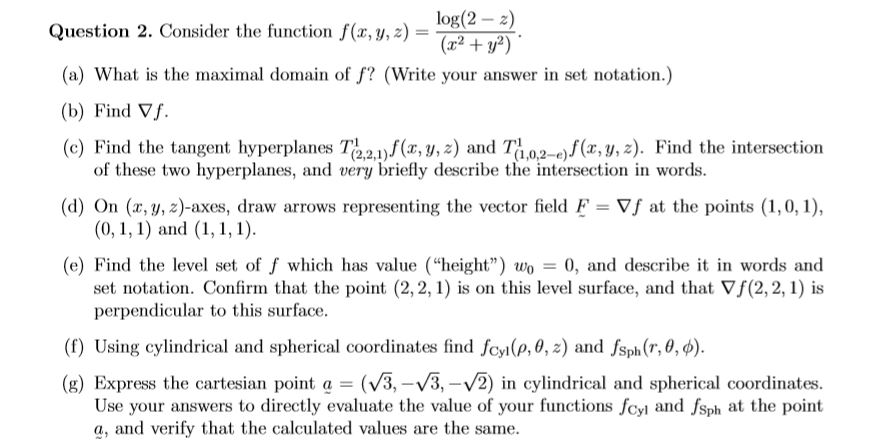log(2 - 2) (x2 y Question 2. Consider the function f(x, y, (a) What is the maximal domain of f? (Write your answer in set notation.) (b) Find ▽f. (c) Find the tangent hyperplnes Te2)(r, y,z) and Tao2-)f(x, y, z). Find the intersection of these two hyperplanes, and very briefly describe the intersection in words (0,1, 1) and set notation. Confirm that the point (2, 2, 1) is on this level surface, and that Vf(2, 2, 1) is (d) On...